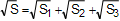Problem 97. Similar Triangles, Areas. Level: High School, SAT Prep, College
 In the figure below, given a triangle ABC, line DEF parallel to AC and line FGM parallel to AB. If S, S1, S2, and S3, are the areas of triangles ABC, DBE, FGE, and MGC respectively, prove that:. View or post a solution.FACTS AND HINTS:

1. COMPARING AREAS OF SIMILAR TRIANGLES:
Proposition:
The areas of similar triangles are to each other as the squares of any two corresponding segments.

Selected Topics

 Home | Geometry | Search | Problems | All Problems | 91-100 | Email | By Antonio Gutierrez